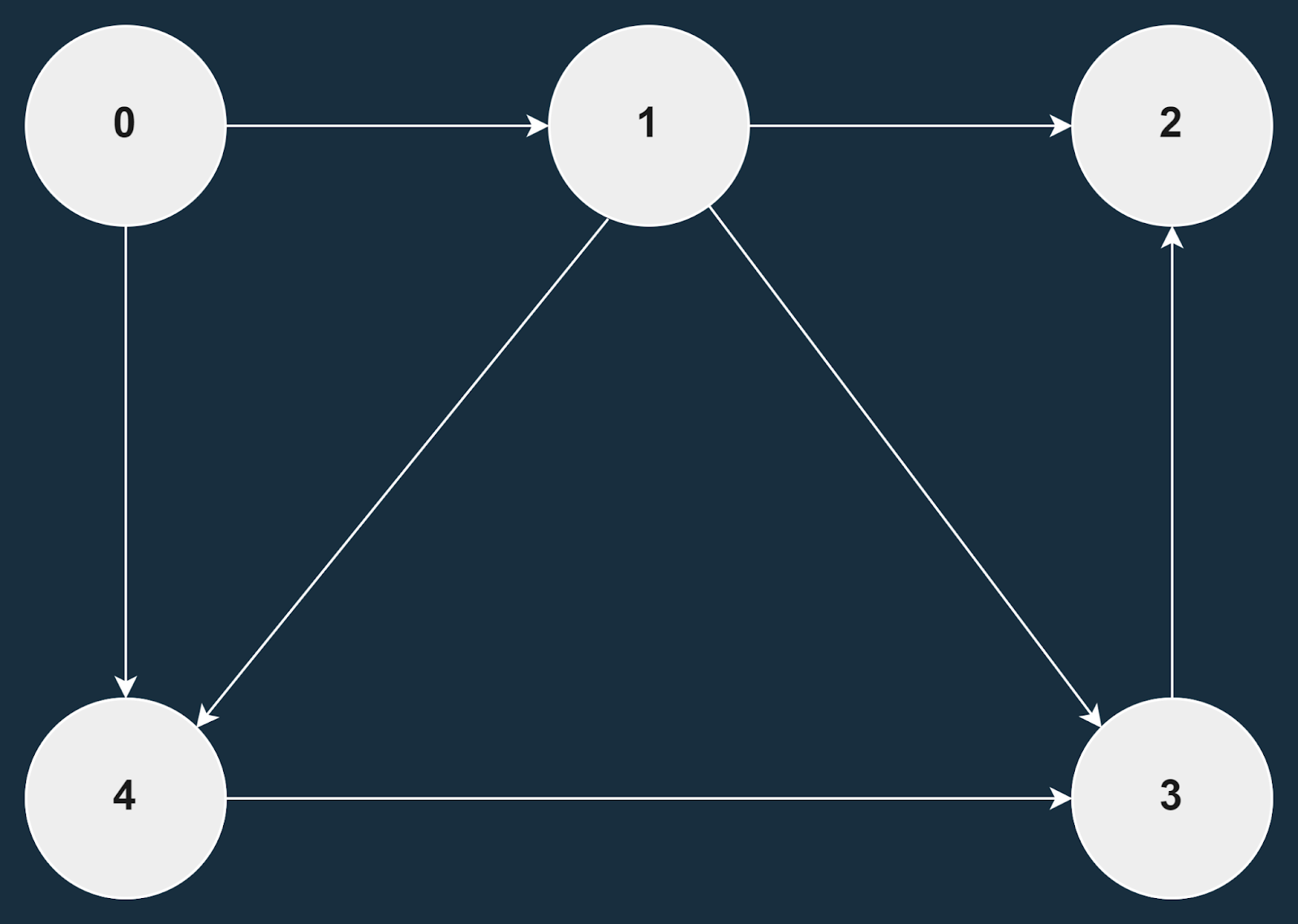# Clone a Directed Acyclic Graph

## Introduction

This blog will discuss the solution to the problem to clone a directed acyclic graph.  Before we deep dive into the solution of this problem, we should look at a sample example to better understand this problem.

### Sample Examples

Input :Output: The cloned graph:

0-1

1-2

3-2

4-3

1-3

1-4

0-4

## Approach

So to solve this problem to clone a directed acyclic graph, we will first do DFS(depth-first search) on the graph. We will take the original node’s value and simultaneously initialize new neighboring nodes with the same value. We will repeat this process till the original graph is fully traversed.

### Implementation in C++

``````// C++ program to clone a directed acyclic graph.
#include <bits/stdc++.h>
using namespace std;

// class for creating a new graph node
class Node
{
public:
int key;

// key is the value of new node
// adj vector will hold a list
// of all the neighbouring nodes
Node(int key)
{
this->key = key;
}
};

// function to print the graph
void printGraph(Node *startNode, vector<bool> &visited)
{
// visiting nodes which are unvisited
{
// looping through the neighbouring nodes of
// the startNode if the source node is not visited
// then print the edge from the source node
// to the neighbouring node after visiting
// all the nodes mark them visited
{
if (!visited[startNode->key])
{
cout << startNode->key <<
"->" << i->key <<
endl;
if (!visited[i->key])
{
printGraph(i, visited);
visited[i->key] = true;
}
}
}
}
}

// function to clone a directed acyclic graph
// we will start traversing the graph depth-wise,
// recursively if we encounter any unvisited node in
// the graph we intialize a new instance of node
// for cloned graph with the original node
Node* cloneGraph(Node *original, Node *newNode, vector<bool> &visited)
{
Node *clone = NULL;

if (!visited[original->key] &&
{
{
// Below check is for backtracking, so new
// nodes don't get initialized everytime
if (clone == NULL ||
(clone != NULL &&
clone->key != originalKey->key))
clone = new Node(originalKey->key);

cloneGraph(originalKey, clone, visited);

// once all the neighbours for the node are
// created in the cloned graph, we will backtrack
// and exit from the node and we will mark the
// node as visited and we will travers next unvisited node
visited[originalKey->key] = true;
}
}

return newNode;
}

// Driver Code
int main()
{
Node *n0 = new Node(0);
Node *n1 = new Node(1);
Node *n2 = new Node(2);
Node *n3 = new Node(3);
Node *n4 = new Node(4);

// to check if the node is visited or not
vector<bool> visited(5, false);
cout << "Graph Before Cloning:-\n";
printGraph(n0, visited);
fill(visited.begin(), visited.end(), false);

Node *clonedGraph = cloneGraph(
n0, new Node(n0->key), visited);

fill(visited.begin(), visited.end(), false);
cout << "\nGraph After Cloning:-\n";
printGraph(clonedGraph, visited);

return 0;
}``````

Output:

``````Graph Before Cloning:-
0->1
1->2
1->3
3->2
1->4
4->3
0->4

Graph After Cloning:-
0->1
1->2
1->3
3->2
1->4
4->3
0->4``````

#### Complexity Analysis

Time Complexity: O(E+V)

The time complexity will be O(E+V) where E is the edges of the graph and V is the vertice of the graph.

Space Complexity: O(1)

The space complexity is O(1).

Q1. What is dfs?

Ans. Dfs or depth-first search is an algorithm for traversing a graph.

Q2. Can there be multiple shortest paths in a graph?

Ans. Yes, we can find multiple shortest paths in a Graph.

Q3. What is a graph?

Ans. A graph is a non-linear data structure consisting of nodes and edges. The nodes can be called vertices, and edges connect two nodes.

## Key takeaways

This article discussed the problem clone a directed acyclic graph and its approach. We hope you have gained a better understanding of the solution to this problem and, now it is your responsibility to solve the problem and try some new and different approaches to this problem.

You can learn more about graphs hereUntil then, Keep Learning and Keep Coding and practicing in Code studio.